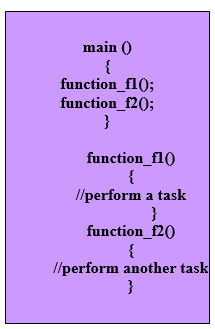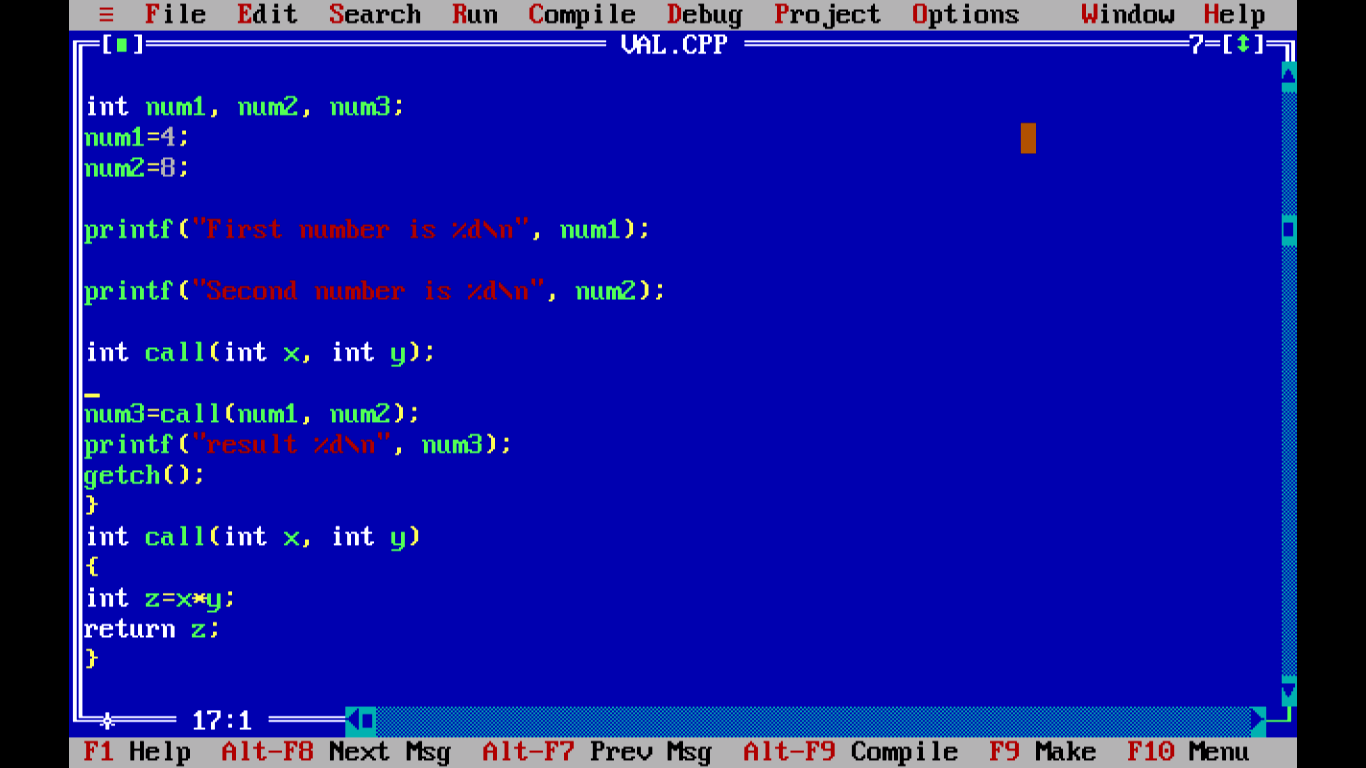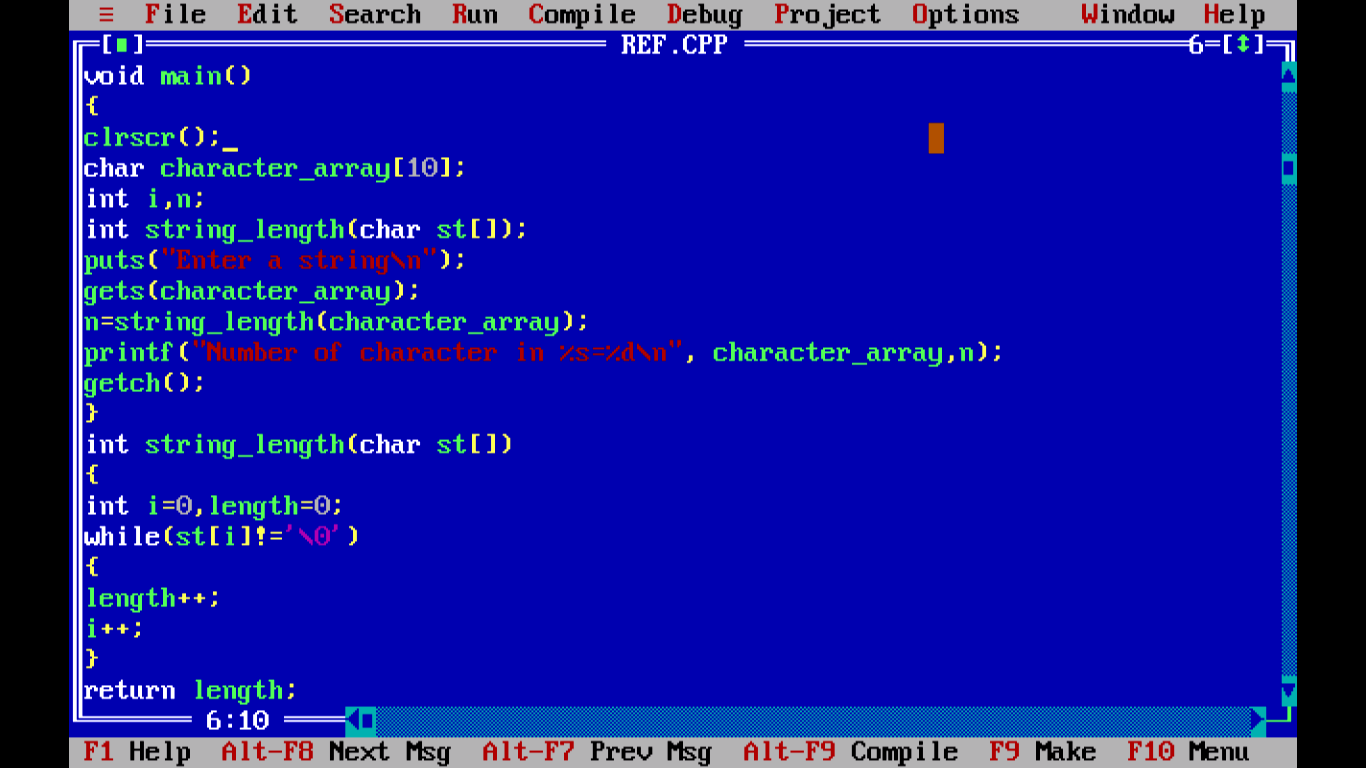# 10 - C Functions

This chapter will introduce functions in C. Functions is one of the important concepts in the programming world. Not only in C language, the concept of functions comes into existence in other programming languages. There are many reasons for having functions. Sometimes it is very difficult to find out logical errors and after finding we need to correct them. When you write an extremely large program you will not know about the errors until and unless you compile the program. Now we have a solution for this problem. We can write some related programs which does some specific task and when they combine together they result in a complete program. These related programs are called modules or functions. Function is a sub program which is responsible for accomplishing a specific task. C program will have at least one function i.e. main() function. There is no limit on the number of functions you define in C program but there can be one and only one main function. The sequence of executing functions depends upon the sequence in which they are specified in main function. As soon as there is no function left for execution, program ends. Following flow diagram will explain the concept.Explanation: In this scenario, execution starts from main() function. First function which it encounters is function function_f1(). Control jumps to function Function_f1() and performs the task specified in this function and the result is returned to main function. In the next sequence of execution in main function, it will encounter second function function_f2(). Control will then jump to function function_f2() and perform the specified task in this function. Then the control will again jump back to main() function. In this way, this program will terminate itself. Let us consider a simple program to explain the mechanism of function.

Example: /*Program to explain functions*/

```# include <stdio.h>
# include <conio.h>
# include <stdlib.h>
# include <math.h>
void subtract;

void main()
{
subtract();
}

void subtract()
{

int a,b,c;
printf("Enter two values\n");

printf("Enter the first number\n");
scanf("%d", &a);
printf("First number is %d\n", a);

printf("Enter the second number\n");
scanf("%d", &b);
printf("Second number is %d\n", b);

c=a-b;

printf("Result is %d\n", c);
getch();
}
```Figure- C  Functions ExampleFigure- C  Functions Example compiled outputFigure- Output of C  Functions example

## 10.1 Types of functions

There are two different types of functions and they are as follows:

1.Built-in functions

2.User-defined functions

### 10.1.1 Built-in functions

Built-in functions are also known as Library functions. These functions are well-defined programs. These programs are general purpose programs. These programs are a part of C compiler itself. These are included in user defined programs which performs a specific and well-defined task. For example,

• sqrt() is a function which finds the square root of a number
• scanf() is a function which accepts the data from keyboard

### 10.1.2 User-defined functions

These are the function which we write. When we write functions, we avoid the need to re-write the code again and again. You write the code once and you can execute it again and again. You can call same function any number of times. There is one more advantage of writing functions and that is it becomes easier to keep track of what are we going to perform. Calling function is the one which is calling another function and called function is the one which is being called. In the above example, main() is the calling function and functions function_f1(), function_f2() are called functions.

## 10.2 Communication between functions

Functions can also pass values. The road to communicate between called and calling function is called arguments or parameters. We have already used them. List of variables and string used inside the parenthesis of printf() function are arguments/parameters.

We have not encountered any program which actually returns a particular value but we will in preceding sections. We have to explicitly specify return keyword in the function if the called function is going to return any result to calling function. As soon as return statement is executed, the control is immediately transferred to calling function. You must be thinking then how we have executed the programs in the previous sections? The answer is we have used void keyword in front of main() function which means it has nothing to accept from any called function. Now there is one more important point to remember and that is a function can return only one value at a time. Let us take a glance on some important concepts.

• Function declaration: We need to declare a function. For example, function_f1() was declared in program. By default, C function returns an integer value but if you want to explicitly return a different value then we need to mention it. Otherwise, compiler will assume it to be of type int.
• Call by value and Call by reference:  This is known as parameter passing. The main difference between these two mechanisms is that in case of call by value mechanism, the value of argument is passed from calling function to called function. If we do any manipulation in the value in called function, no change will appear in calling function.

Example: /* Program to illustrate call by value*/

```# include <stdio.h>
# include <conio.h>
# include <stdlib.h>
# include <math.h>

void main()
{
clrscr();
int num1, num2, num3;
num1=4;
num2=8;
printf("First number is %d\n", num1);
printf("second number is %d\n", num2);
int call(int x, int y);
num3=call(num1,num2);
printf("Result %d\n", num3);
getch();
}
int call(int x, int y)
{
int z=x*y;
return z;
}
```

Snapshots of example programs:Figure – C Call by Value exmpleFigure – Compiled output of C call by value ExampleFigure - Output of Call by value example program

Call by reference is a method which passes address to calling function instead of actual values. Memory locations are referred here, not the values. In contrast to called by value, if there is any change made to value in called function then this change is also reflected in calling function. You must be thinking what is this address? The answer to this Pointers. We have a dedicated chapter for explaining pointers. It is one of important and complex concept in C language. Following program will illustrate the scenario of call by reference.

Example: /* Program to illustrate call by reference */

```# include <stdio.h>
# include <conio.h>
# include <stdlib.h>

void main()
{
clrscr();
char character_array;
int i,n;
int string_length(char st[]);

puts("Enter a string\n");
gets(character_array);
n=string_length(character_array);

printf("Number of characters in %s=%d\n", character_array,n);
getch();
}

int string_length(char st[])
{
int i=0, length=0;
{
length++;
i++;
}
return length;
}
```Figure – C Call by reference exampleFigure – C Call by reference example compiled outputFigure - Output of C Call by reference example

• Recursion: This is properly known as function calling itself. In this scenario, function is defined in terms of itself. This sort of function performs a task repeatedly by calling itself. There is a very important point to remember about this mechanism is that there has to be an exclusive terminating condition, otherwise function will go into a never ending loop. Recursion is elaborately explained in a separate chapter in later section.

We are concluding this chapter. Next chapter is dedicated to C pre-processors. Thank you.